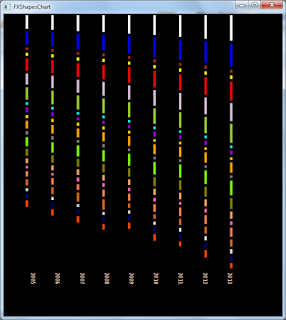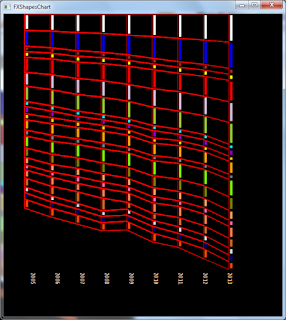### Javafx Shapes("Revenue DataViz")

As per usual new data uploaded on kenyaopendata,means more javafx exploration with data,and this time with only Shapes i.e Polygon,Lines.Rectangle.

My first problem was to find how to best scale Shapes ,thanks to the book Beautiful Visualization by Julie Steele and Noah lliinsky,i found a formula that fits best although i tried a couple of others.

The formula in java

Given that the large value is represented by 50 pixels along Y axis
finding the size of other value will be

double lengthY=Math.sqrt(Math.pow(50,2)*target_value)/MAX_VALUE

Drawing the Lines

The idea was to draw a line from StartX,StartY add the lengthY  to EndY plus a value for spacing for the next line, giving an illusion of a broken,below its an example of how i did it.

int temp=5;
Y_OFFSET=11;

for(int i=0;i<5;i++){

Line line=new Line();
line.setStrokeWidth(5);
line.setStroke(Color.RED);

line.setStartX(5);
line.setStartY(temp);

line.setEndX(5);
line.setEndY(lengthY+temp);

temp=line.getEndY()+Y_OFFSET;

temp+=5;
}

Since the data has data for 9 years i had to create a for loop within a for loop the outer loop for x_axis and inner loop for y_axis.

for(int j=0;j<9;j++){
for(int i=2;i<23;i++){

double d=Math.sqrt(Math.pow(50,2)*target_value/MAX_VAL);

Line line=new Line();
line.setStrokeWidth(5);

line.setStroke(Color.RED);
line.setStartX(x_axis);
line.setStartY(temp);

line.setEndX(x_axis);
line.setEndY(d+temp);
temp=line.getEndY()+Y_OFFSET;

}
x_axis+=55;//move 55 pixels along X axis
}
Creating the polygon
We have the lines and they are all given ids "line.setId()" or categories ,so as to be able to find all the nodes with the same ID

List<Double> points=new ArrayList();
List<Double> points1=new ArrayList();

for(Node n:root.lookupAll("#"+idName)){
Line l=(Line) n;
}
Collections.reverse(points);//the polygon follows the points round

We are done,only styling now.
The stages....Color coded in CategoriesTransparent fillLine transparent Polygon fill